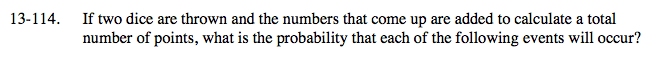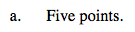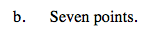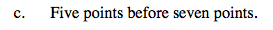### Home > A2C > Chapter 13 > Lesson 13.2.2 > Problem13-114

13-114.
1. If two dice are thrown and the numbers that come up are added to calculate a total number of points, what is the probability that each of the following events will occur? Homework Help ✎

1. Five points.

2. Seven points.

3. Five points before seven points.$\frac{4}{36}=\frac{1}{9}$$\frac{6}{36}=\frac{1}{6}$How many events are important?

Of these, how many give the desired result?